-->

Melihat Dunia Dengan Data Sebagai Sebuah Investasi

# ANALYSIS IMDB USING R

Good evening, this time atmosphere of jakarta just as typically "STUCK" so to take advantage of a little bit of free time after work and then now I am going to write about the IMDb (Internet Movie Database) in order for us to learn.

Okay straight to the topic of our discussion.

I have the IMDb data from ranges of years 1920-2016.
# Import Data .csv
# Packages
library(ggplot2)
library(plotly)
library(dplyr)
library(magrittr)
library(corrplot)
library(corrr)
library(ggthemes)

Advisable that you first checking against data sets before exploration.
In this case:
I noticed that there were some duplicates in the data set. In some cases, every bit of information was the same apart from a measure such as number of user votes being different by 1. I deleted any duplicate lines based on the movie title.
#Remove duplicates based on name

Movie.Data <- movie[!duplicated(movie\$movie_title),]

# **How would you describe the net profit of each film in 1920-2015 according to the data?**

First authors will see the distribution of the film production company will be divided into 9 decades from the entire data set. In order to view the description of the production of movies based on existing data. It is used to facilitate us in describing the advantages of each film.
#functions to find value of the frequency
frek=function(x,y,z){
a=0
for(i in 1 : length(movie\$title_year)){
if(x[i]>=y && x[i]<=z){
a=a+1}}
print(a)}
#call the function to frequency values per decade
frek (movie\$title_year, 1920, 1936)
frek (movie\$title_year, 1937, 1946)
frek (movie\$title_year, 1947, 1956)
frek (movie\$title_year, 1957, 1966)
frek (movie\$title_year, 1967, 1976)
frek (movie\$title_year, 1977, 1986)
frek (movie\$title_year, 1987, 1996)
frek (movie\$title_year, 1997, 2006)
frek (movie\$title_year, 2007, 2016)
#the order to form the frequency table
tabel=edit(data.frame( ))
#plot
g_movie= ggplot(data =tabel, aes(y=tabel\$Frequence, x=tabel\$`Year Of Movie Production`, fill=tabel\$`Year Of Movie Production`));
g_movie + geom_bar(stat = "identity", width = 0.2, position = "identity") +
xlab("Decades") + ylab("Number of movies") +
ggtitle("Frequency of movies by decades") +
theme(axis.text.x = element_text(angle = 45, hjust = 1));

The above results suggest that the production of the movies of the Decade every increasing distribution forms, such as ekponensial. A very rapid increase in visible from 1996 to 2016.
Perhaps this due to technological advances in the field of cinema. The author is also interested in finding out about the performance of the film in the years before 1996, author will use to compare the performance of the film in the years thereafter. Performance will be judged on the level of ROI generated each film.
Okay, my steps to describe the ROI are ekplorasi:
step one:
"I add fields for the profit and return on investment (ROI)"
Okay, maybe my first suggest that reading about how to calculate profit and ROI in some journal or book economics.
profit formula is as follow:
**Profit = gross - budget**
ROI can also be defined as the ratio of net profits against the cost. Calculate ROI formula is as follows:
**ROI = (profit/budget) x 100%**
Why do authors use value ROI?
Because, Return on Investment (ROI) is the benefit to an investor resulting from an investment of some resource. A high ROI means the investment gains compare favorably to investment cost. As a performance measure, ROI is used to evaluate the efficiency of an investment or to compare the efficiency of a number of different investments.
# adding two colums: profit and percentage return on investment.
Movie.Data=Movie.Data %>%
mutate(profit = gross - budget,
ROI = (profit/budget)*100)
After we calculate ROI, then let’s look at the earnings of each film with visualization approach.
Q=plot_ly (Movie.Data, x = ~title_year, y = ~ROI, text = ~movie_title,
type = 'scatter', mode = 'markers',
size = ~ROI, color = ~country, colors = 'Paired',
marker = list(opacity = 0.5, sizemode = 'diameter'))%>%
layout(title = 'Return On Investment Movie Production 1920-2016',
xaxis = list(showgrid = FALSE),
yaxis = list(showgrid = FALSE),
showlegend = FALSE)
Q

Results visualization above indicates that there are 4 movies with very high income, i.e.,:
1. Law Abiding Citizen (719348.553 %) (year = 2007)
2. Cloudy with a Chance of Meatballs (271466.055 %) (year = 2003)
3. Edmond (234116.857 %) (year = 1999)
4. Sausage Party (159130.990 %)(year = 1997)
The average revenue from each movies is of:
mean(Movie.Data\$ROI)
##  -99.9982
Value of minimum income from each film is of:
min(Movie.Data\$ROI)
##  719348.6

If viewed from the range of earnings of each film, the minimum value of profits show that there movies that suffered losses.
thus formed new data frames based on value of minimum and maximum ROI, i.e.,

#movies that have benefited
Movie.Data.profit <- Movie.Data %>%
arrange(desc(ROI)) %>%
filter(ROI >= -0.0)
#movie that gets the loss
Movie.Data.profit.Low <- Movie.Data %>%
arrange(desc(ROI)) %>%
filter(ROI < 0.0)
length
(Movie.Data.profit\$ROI)
##  2506
There is as much as 2506 the movie does not suffer losses.
then consider the following graph:

z = plot_ly(Movie.Data.profit, x = ~title_year, y = ~ROI, text = ~movie_title,
type = 'scatter', mode = 'markers',
size = ~+ROI, color = ~country, colors = 'Paired',
marker = list(opacity = 0.5, sizemode = 'diameter'))%>%
layout(title = 'The movie with surplus income in year 1920-2016',
xaxis = list(showgrid = FALSE),
yaxis = list(showgrid = FALSE),
showlegend = FALSE)
z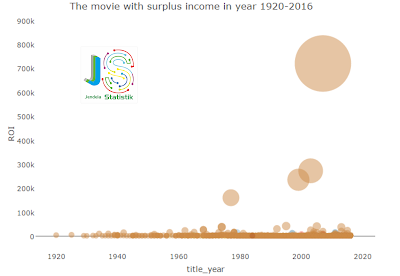The above results indicate that quantity value of the Return on investment of every movie between the value 0.0 - 719.3486 %. And then the author will see how the value of the loss of each film from 1990-2016.
length (Movie.Data.profit.Low\$ROI)
##  2345
And then there are as many as 2345 the film loss.
then consider the following graph:
w = plot_ly(Movie.Data.profit.Low, x = ~title_year, y = ~ROI, text = ~movie_title, type                      = 'scatter', mode = 'markers',
size = ~-ROI, color = ~country, colors = 'Paired',
marker = list(opacity = 0.5, sizemode = 'diameter'))%>%
layout(title = 'The movie suffered a loss in year 1920-2016',
xaxis = list(showgrid = FALSE),
yaxis = list(showgrid = FALSE),
showlegend = FALSE)
w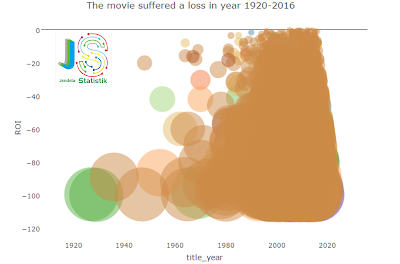Results visualization above shows an indication of the huge losses beginning in 1927 by film “My Dog Tulip” up to huge losses each film undergoing a phase of very large spike in numbers on a span of 1969-2016, ranging between -0.0682600 - -99.99820 % Return on investment.
Later, the author will be compare the number of films that are experiencing losses and profit in the range of years 1996-2016. It is used to assess the performance of films produced, i.e.,

#number of films that fortunately
frek.p=function(x,y,z) {a=0
for(i in 1 : length(Movie.Data.profit\$title_year)){
if(x[i]>=y && x[i]<=z){
a=a+1}}
print(a)}
#Call function
frek.p (Movie.Data.profit\$title_year, 1996, 2016)
##  1881
The number of films that benefit based on the value of ROI on range 1996-2016 is as much film 1881.
While the movies suffered losses based on the value of ROI in the year range is as much as 2171 movies.
#the number of film loss
frek.r=function(x,y,z) {
a=0
for(i in 1 : length(Movie.Data.profit.Low\$title_year)){
if(x[i]>=y && x[i]<=z){
a=a+1}}
print(a)}
#Call function
frek.r (Movie.Data.profit.Low\$title_year, 1996, 2016)
##  2171
The above results indicate that the performance of films produced in the span of 1996-2016 is not so good in generating profits. In order to make sure it let’s see calculation of film experience gains and losses before 1996.
The number of films that are experiencing the advantage on a range of 1995-1920, i.e,
frek.p (Movie.Data.profit\$title_year, 1920, 1995)
##  625
The number of films that are experiencing losses in the span of 1995-1920, i.e,
frek.r (Movie.Data.profit.Low\$title_year, 1920, 1995)
##  174
The results above show that in the year before the 1996 performance of films produced a pretty good advantage. It can be seen from as many as 625 film or revolves around 3 times the amount of movies that suffer losses i.e. 174 movie.

conclusion
Film production increased every decade in terms of number of films. But film produced in 1996-2016 many experiencing a deficit. While the number of films that are having difisit on production prior to 1996 are much less. This indicates that the film before the year 1996 has good performance in generating ROI for investors.

# **Based on the data above, make an order of the film category (content_rating) from the most liked film category based on imdb_score! Give your explanation!**

Before continue analysis the author find out about categories of content ratings that are elaborated as follows:
G: General
The film is safe to be witnessed by all ages, including children.
PG: Parental Guidance
This movie was recommended to be seen with supervision and mentoring.
PG-13: Parental Guidance Above 13
This movie is very recommended mentoring and supervision of extra parents.
R: Resticted
The film is to look further to be seen by children up to the age of adolescence.
NC-17: No One 17 and under Admitted
The film was banned to see kids to teenagers who are not yet 18 years old.
M (Mature)
This program is specifically designed to be viewed by adults and therefore may be unsuitable for children under 17.
approved
This program is designed to be appropriate for all children.
TV-14 : Parents Strongly Cautioned
This program contains some material that many parents would find unsuitable for children under 14 years of age.
TV-G : General Audience
Most parents would find this program suitable for all ages.
TV-MA :Mature Audience Only
This program is specifically designed to be viewed by adults and therefore may be unsuitable for children under 17.
TV-PG : Parental Guidance Suggested
This program contains material that parents may find unsuitable for younger children. Many parents may want to watch it with their younger children.
Unrate
Unfinished given content category ratings but has been given permission to broadcast.
X
films are restricted to adults. This classification is a special and legally restricted category which contains only sexually explicit content.
reference:

Then, author tried to see the overview of content of movie most preferred categories based on the imdb score. Here is the plot which describe conditions that:
#plot content based on imdb Score
plot_ly(Movie.Data, x = ~content_rating, y = ~imdb_score, size = ~imdb_score,
color = ~content_rating, colors = 'Paired',
text = ~movie_title,type = 'scatter', mode = 'markers',
marker = list(opacity = 0.5, sizemode = 'diameter')) %>%
layout(title = 'film content distribution based on imdb_score',
xaxis = list(title = FALSE),
yaxis = list(showgrid = FALSE),
showlegend = FALSE)The above results suggest that the categories of content based on the imdb score look almost the same distribution form. So, then the author will do the exploration of content rating and imdb score based on other variables where they have a role in determining the formation of value imdb.
To looking for patterns of relationships of any variable that is expected to have an impact on the value of imdb_score can use a simple trick i.e. with making the correlation between imdb_score bar plot with each data set.
#new data frame
Movie_RM_NA <- movie
Movie_RM_NA %<>% remove_missing()
#select numeric columns
nums <- sapply(Movie_RM_NA, is.numeric)
#and here you have "the most important correlations" for variable cases excluding character variables
Movie_Corr <- Movie_RM_NA[,nums] %>% correlate() %>% focus(imdb_score)                                                                       %>%  filter(imdb_score > 0.0 | imdb_score < -0.0)
#plot
Bar_Corr <- ggplot(data=Movie_Corr, aes(x=rowname, y=imdb_score)) +
geom_bar(stat="identity", position="identity", fill = "darkturquoise") +
theme_solarized(light=FALSE) +
scale_colour_solarized("red") +
ylab("Correlations") +
xlab("Correlation Variables") +
ggtitle("Greatest Correlations between IMDB and Other Variables") +
coord_flip()
ggplotly(Bar_Corr)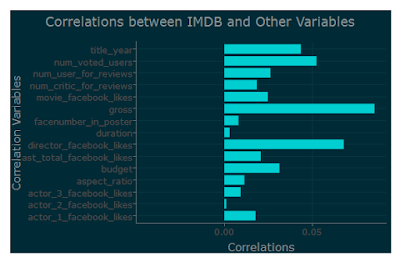There are three variables if seen statistically based on the value of the correlation tables that are formed i.e. gross, the num voted users, director of facebook likes.
However, gross variable according to the author more influential towards mapping out the profit. So, the author uses two variable num voted user and facebook director likes as an indicator.
y <- plot_ly(Movie.Data, x = ~content_rating, y = ~imdb_score,size =                           ~num_voted_users,
color = ~content_rating, colors = 'Paired',
text = ~movie_title,type = 'scatter', mode = 'markers',
marker = list(opacity = 0.5, sizemode = 'diameter')) %>%
layout(title = 'film content distribution based on imdb_score and num_voted_users',
xaxis = list(title = FALSE),
yaxis = list(showgrid = FALSE),
showlegend = FALSE)
y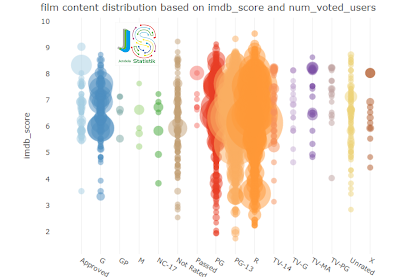Results showed that the content of the film is much liked by the public is PG, PG-13, R, G. But the author only take three categories the most widely preferred film based on the value of the num voted for the user. And then formed the plot back to be able to see the category of content where the most-preferred based on num voted user.
#reduction of the amount of content
Movie.Data\$content_rating <- as.factor(c('PG', 'PG-13', 'R'))
#plot Top 3 Content rating
o <- plot_ly(Movie.Data, x = ~content_rating,
y = ~imdb_score,size = ~num_voted_users,
color = ~content_rating, colors = 'Paired',
text = ~movie_title,type = 'scatter', mode = 'markers',
marker = list(opacity = 0.5, sizemode = 'diameter')) %>%
layout(title = 'Top 3 content rating based on imdb_score and num_voted_users',
xaxis = list(title = FALSE),
yaxis = list(showgrid = FALSE),
showlegend = FALSE)

o
The results above show that film for the category content PG-13 (Parental Guidance Suggested = “Some of the content in the film may be inappropriate for viewing for Children Under age 13”) the most preferred is the film “A Turtle’s Tale: Sammy’s Adventures” and the film is the most preferred content category based on the num voted user.
And based on the visualization above, seen that movie with a category content PG-13 is the content categories with the num voted for the user most high. It also indicated that categories of content rating PG-13 is the most preferred by the user among other content categories rating.
After that, the authors see the preferred content category based on Director facebook likes.
#new data frame
Movie_dir <- movie
Movie_dir %<>% remove_missing()
#plot
D <- plot_ly(Movie_dir, x = ~content_rating, y = ~imdb_score,size =               ~director_facebook_likes,
color = ~content_rating, colors = 'Paired',
text = ~movie_title,type = 'scatter', mode = 'markers',
marker = list(opacity = 0.5, sizemode = 'diameter')) %>%
layout(title = 'film content distribution based on imdb_score and                    director_facebook_likes',
xaxis = list(title = FALSE),
yaxis = list(showgrid = FALSE),
showlegend = FALSE)
D

Results showed the three categories of content PG, PG-13, R is the most preferred rating content according the Director facebook like. In order to see the results of more detailed then the author makes the plot of three categories of such content.
#reduction of the amount of content
q=Movie_dir\$content_rating <- as.factor(c('PG', 'PG-13', 'R'))
#plot Top 3 Content rating
Dir <- plot_ly(Movie_dir, x = ~content_rating,
color = ~content_rating, colors = 'Paired',
text = ~movie_title,type = 'scatter', mode = 'markers',
marker = list(opacity = 0.5, sizemode = 'diameter')) %>%
layout(title = 'Top 3 content rating based on imdb_score and director_facebook_likes',
xaxis = list(showgrid = FALSE),
yaxis = list(showgrid = FALSE),
showlegend = FALSE)
Dir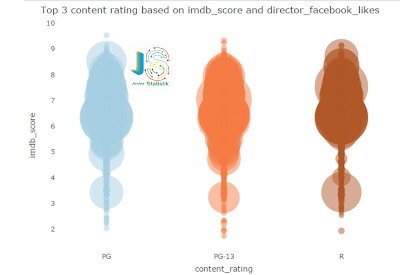The above results show that these three categories form a similar data distribution. This may be due to the Director of a film category with other content categories also works in the making of the film with different content categories.

# conclusion:

the above results indicate that three film categories content i.e. PG, PG-13, R based on the imdb score, num voted user and Director facebook likes are the three most preferred content.

Okay, enough up here used to be Yes learn ours. another time we will continue again. see you again ...

Best Regards